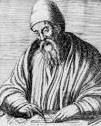## Monday, July 30, 2007

### Creative Approach 有什麼不同 (2) - 談談數學歸納法

Udi Manber 在他的裡第二章末，參考文獻與進階讀物一節裡提到，數學歸納法是由 Francesco Maurolico 所發展出來的，事實上，數學歸納法的基礎可以上溯至更早的希臘時代的歐幾里德Euclid），維基百科可以找到數學歸納法的歷史介紹，簡明扼要但資料豐富，有意者可自行深入研究。

• P(0) 成立 (有的情況是講P（1）)
• 當 P(n) 成立，則 P(n+1) 也成立

• The basis: P(0) is true.
• The inductive step: If p(n) holds, then P( n + 1) holds too.

1. P(0)
2. For all n ≥ 0, P(n) ⇒ P(n + 1)

For all m ≥ 0, P(m) is true ...................................... （3）

1. 0是一個自然數。
2. 若x是一個自然數，則另有一個自然數X'(緊隨X之後)。X'稱為X的直接繼數。
3. 0不是任何數的後繼者
4. 對任何自然數X與y，若X'=Y'，則X=Y。
5. [歸納原理]若P是一個性質(Property)，並且(i)0其有性質P；(ii)若任何自然數X具有性質P：則X'具有性質P，則所有自然數其有性質P。

• The Base Case (in the domino analogy, this shows the first domino will fall)
• The Induction Hypothesis (in the domino analogy, we assume that a particular domino will fall)
• The Inductive Step (in the domino analogy, we prove that the domino we assume will fall will cause the next domino to fall)

☆（１）先證明ｎ＝１，ｎ＝２時命題成立；

（２）假設ｎ＝ｋ－１，ｎ＝ｋ－２的時候命題成立

（３）證明在此情形下，ｎ＝ｋ時命題也成立。

☆（１）先證明ｎ＝１到ｍ時命題成立；

（２）假設ｎ＝ｋ的時候命題成立

（３）證明在此情形下，ｎ＝ｋ＋ｍ時命題也成立。

Standard Formulation

• The basis: P(0) is true.
• Induction hypothesis: assume P(n=m) holds.
• The inductive step: If p(m) holds, show that P( m + 1) holds too.

Strong Induction

• The basis: P(0) is true.
• Induction hypothesis: assume for all n <= m, P(n) holds.
• The inductive step: If p(n) holds, show that P( n + 1) holds too.

Reverse Induction

• P(M) is true for some M
• If P(n=k+1) holds, show that the property holds for n = k.

Transfinite Induction

Transfinite induction 一般而言是應用在 well-ordered set 上，通常只有真正念數學的人，才會關心這個咚咚吧？ 根據 PlanetMath 的說明， Transfinite induction 的定義如下：#### 1 comment:

1.謝謝...
你這篇關於 Principle of M.I. 的整理, 獲益良多.

### 如果我的心是一朵蓮花

~ 林徽因 · 馬雁散文集 · 蓮燈 ~ 馬雁 在她的散文《高貴一種，有詩為證》裡，提到「十多年前，還不知道林女士的八卦及成就前，在期刊上讀到別人引用的《蓮燈》」 覺得非常喜歡，比之卞之琳、徐志摩，別說是毫不遜色，簡直是勝出一籌。前面的韻腳和平仄的處理顯然高於戴...